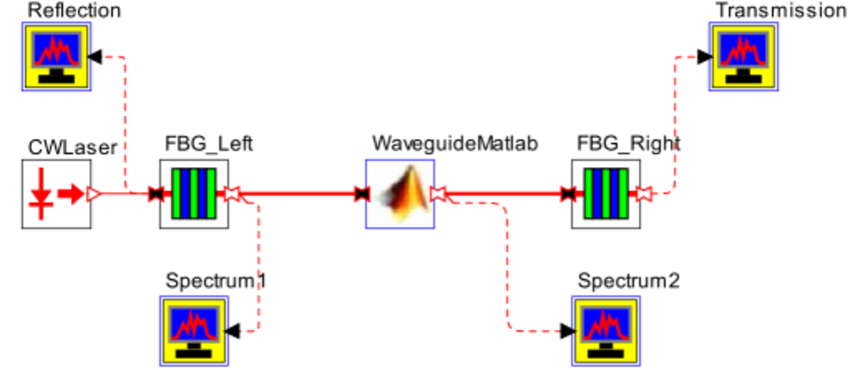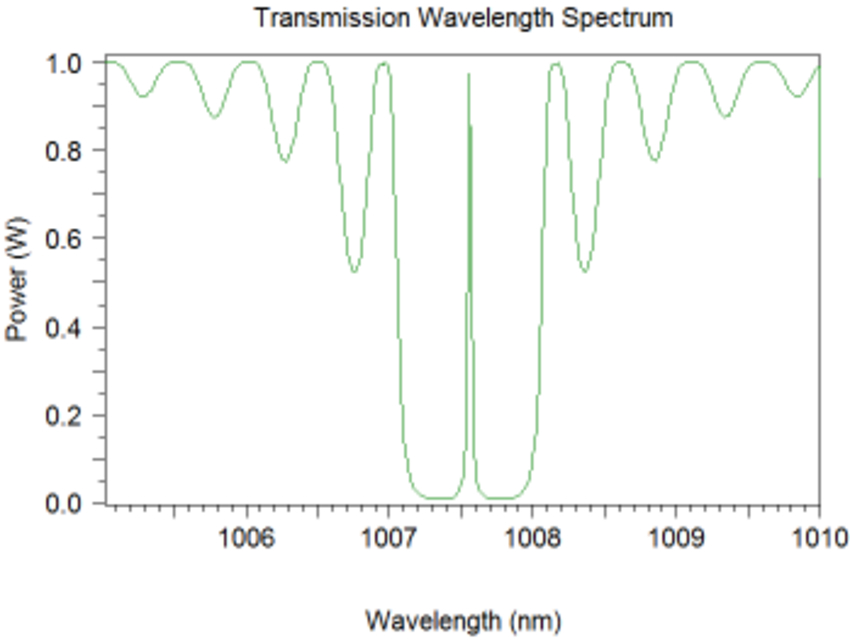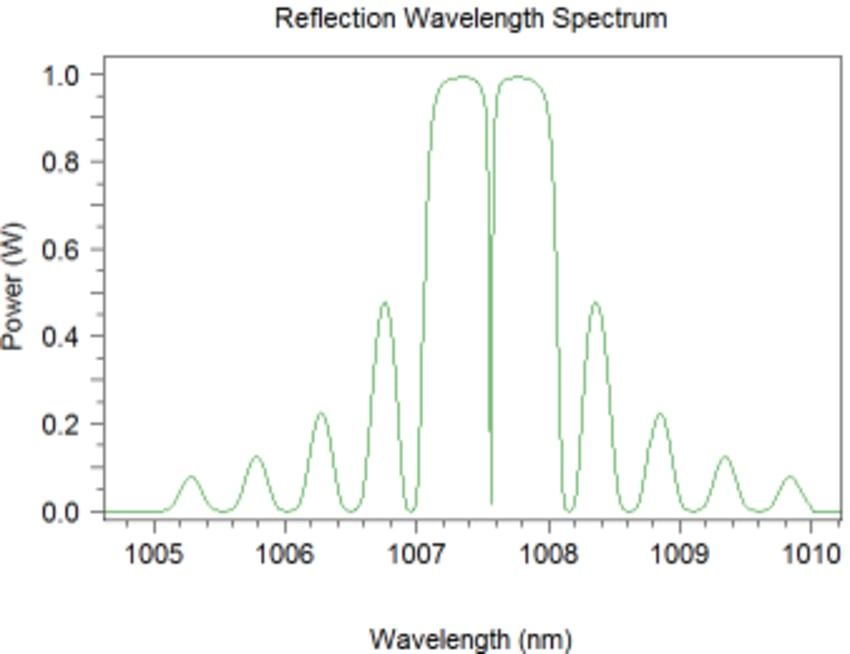# Modeling Custom Photonic Integrated Circuit (PIC) Elements Using OptSim Circuit Cosimulation with MATLAB

## Tool Used: OptSim Circuit

As the push towards photonic integration intensifies, designers want flexibility to develop proprietary intellectual property (IP) and model custom PIC elements via cosimulation with the third-party tools such as MATLAB. With OptSim Circuit, it is possible to use cosimulation with MATLAB to model custom bidirectional PICs and PIC elements. As an illustration, the layout in Figure 1 depicts a notch filter PIC1 with a custom waveguide modeled in MATLAB.Figure 1: OptSim Circuit cosimulation with MATLAB

During simulation, OptSim Circuit invokes MATLAB, which executes the MATLAB source code for the custom model.

MATLAB simulation results are then passed to OptSim Circuit for the remaining modeling tasks. For the notch filter PIC schematic of Figure 1, the transmission and reflection spectra are shown in Figure 2.Figure 2: Transmission (a) and reflection (b) spectra for the notch filter PIC